Скачать презентацию 5 1 Write Linear Equations in Slope-Intercept Form

ca950739789a86c1c10fa9356857b212.ppt

• Количество слайдов: 19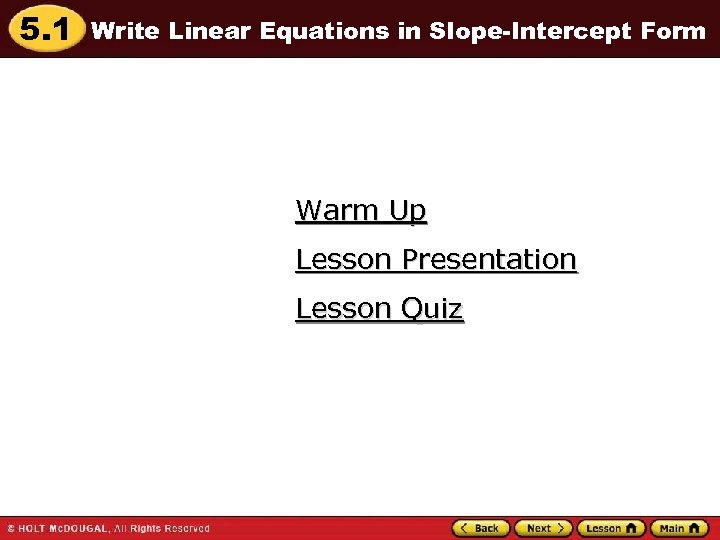5. 1 Write Linear Equations in Slope-Intercept Form Warm Up Lesson Presentation Lesson Quiz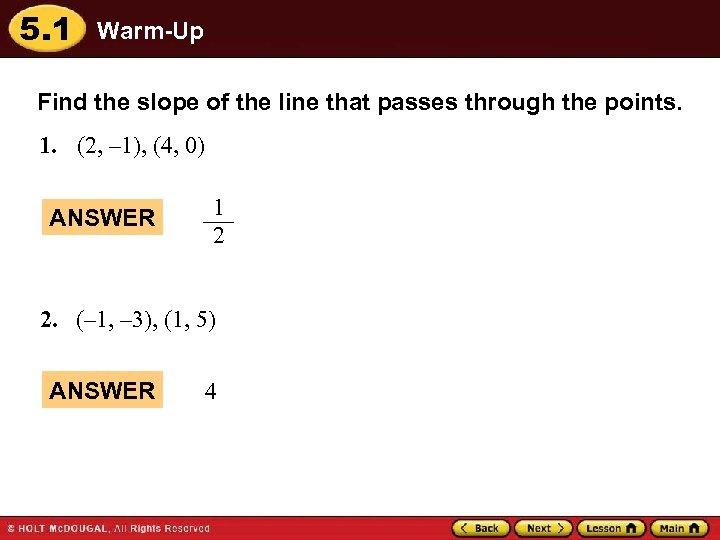5. 1 Warm-Up Find the slope of the line that passes through the points. 1. (2, – 1), (4, 0) ANSWER 1 2 2. (– 1, – 3), (1, 5) ANSWER 4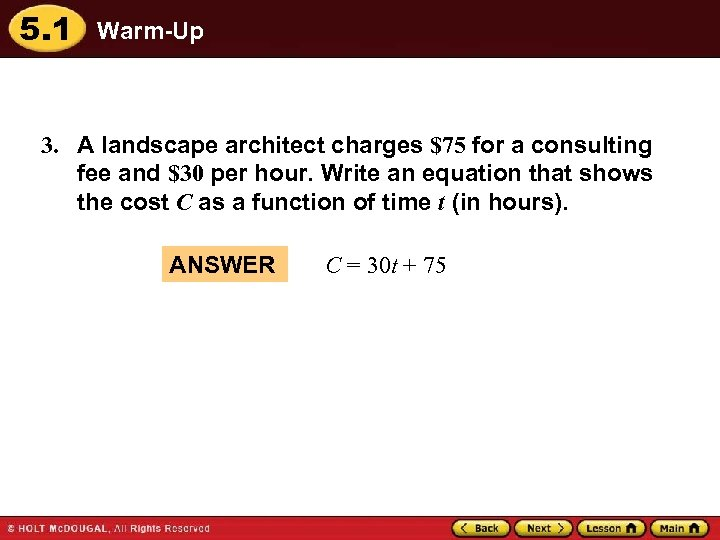5. 1 Warm-Up 3. A landscape architect charges \$75 for a consulting fee and \$30 per hour. Write an equation that shows the cost C as a function of time t (in hours). ANSWER C = 30 t + 75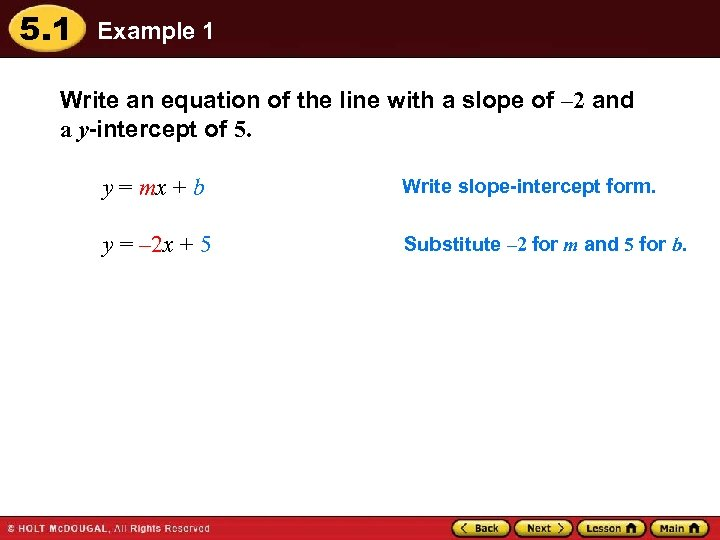5. 1 Example 1 Write an equation of the line with a slope of – 2 and a y-intercept of 5. y = mx + b Write slope-intercept form. y = – 2 x + 5 Substitute – 2 for m and 5 for b.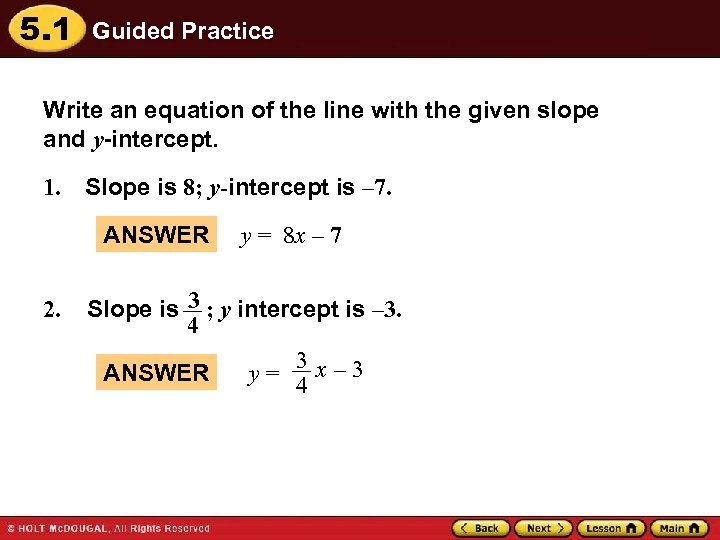5. 1 Guided Practice Write an equation of the line with the given slope and y-intercept. 1. Slope is 8; y-intercept is – 7. ANSWER 2. y = 8 x – 7 Slope is 3 ; y intercept is – 3. 4 3 x– 3 ANSWER y= 4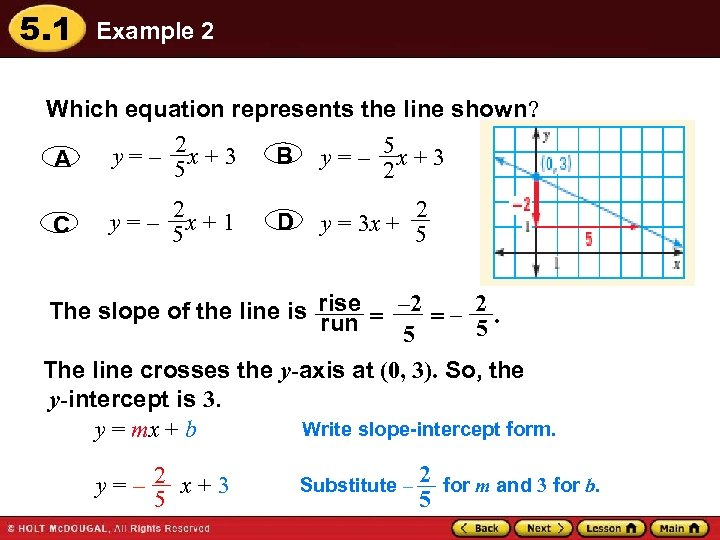5. 1 Example 2 Which equation represents the line shown? 2 B y = – 5 x + 3 y=– x+3 A 5 2 C y=– 2 x+1 5 D 2 y = 3 x + 5 The slope of the line is rise = – 2. run 5 5 The line crosses the y-axis at (0, 3). So, the y-intercept is 3. Write slope-intercept form. y = mx + b y=– 2 x+3 5 Substitute – 2 for m and 3 for b. 5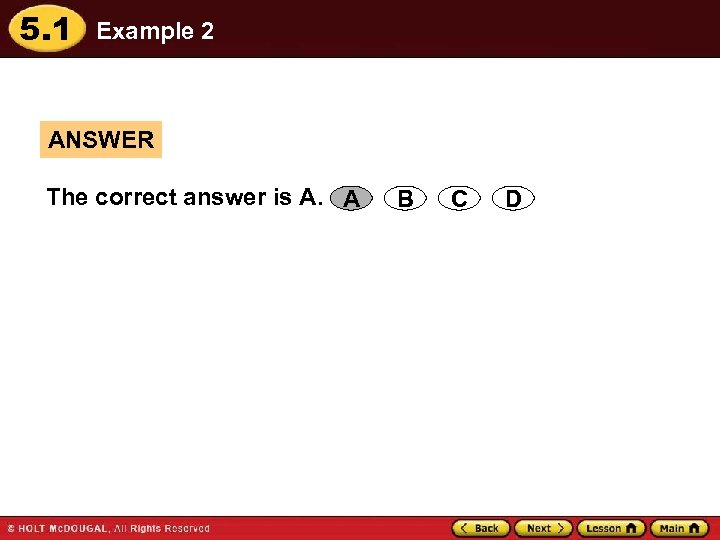5. 1 Example 2 ANSWER The correct answer is A. A B C D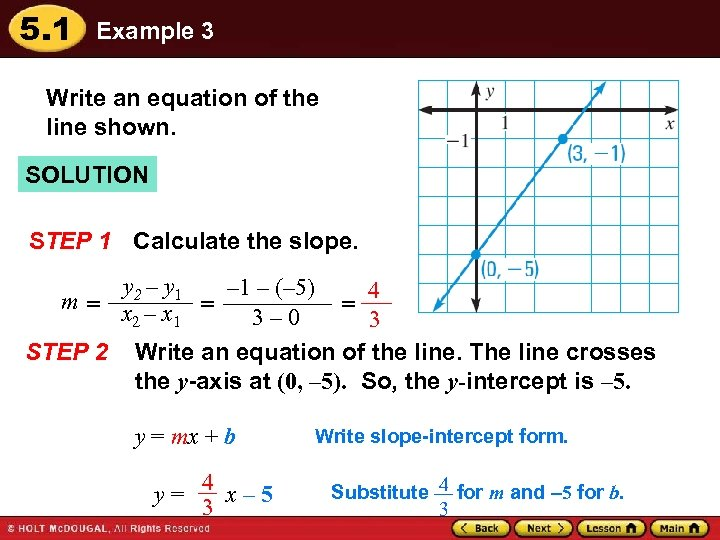5. 1 Example 3 Write an equation of the line shown. SOLUTION STEP 1 Calculate the slope. y 2 – y 1 – (– 5) 4 m = x –x = = 3– 0 2 1 3 STEP 2 Write an equation of the line. The line crosses the y-axis at (0, – 5). So, the y-intercept is – 5. y = mx + b y= 4 x– 5 3 Write slope-intercept form. Substitute 4 for m and 5 for b. 3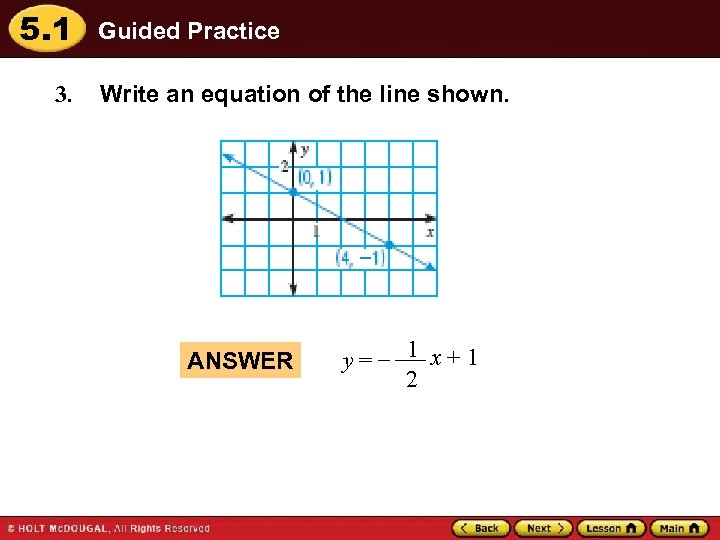5. 1 3. Guided Practice Write an equation of the line shown. ANSWER y=– 1 x+1 2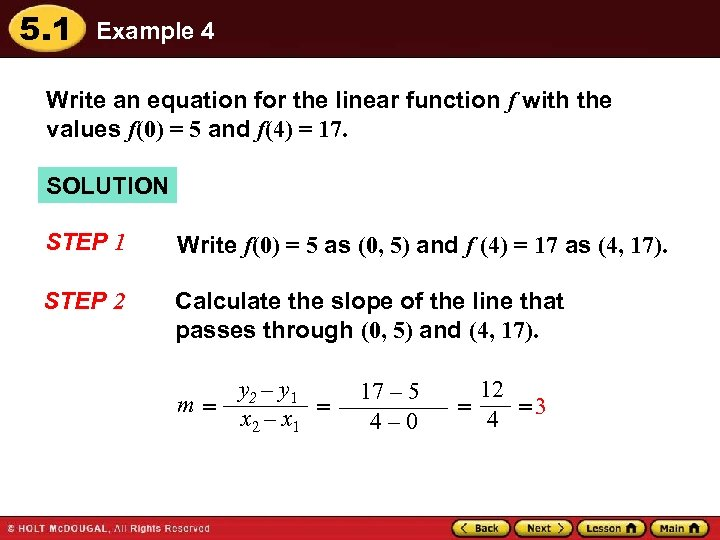5. 1 Example 4 Write an equation for the linear function f with the values f(0) = 5 and f(4) = 17. SOLUTION STEP 1 Write f(0) = 5 as (0, 5) and f (4) = 17 as (4, 17). STEP 2 Calculate the slope of the line that passes through (0, 5) and (4, 17). y 2 – y 1 m= x 2 – x 1 = 17 – 5 4– 0 12 = 4 =3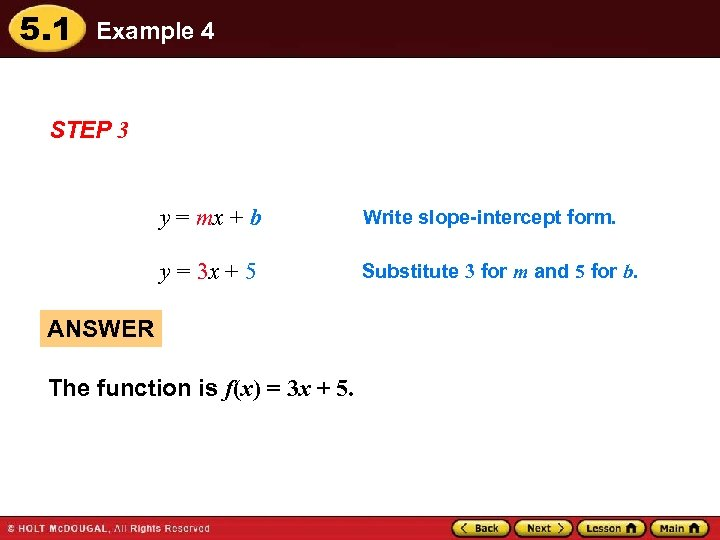5. 1 Example 4 STEP 3 y = mx + b Write slope-intercept form. y = 3 x + 5 Substitute 3 for m and 5 for b. ANSWER The function is f(x) = 3 x + 5.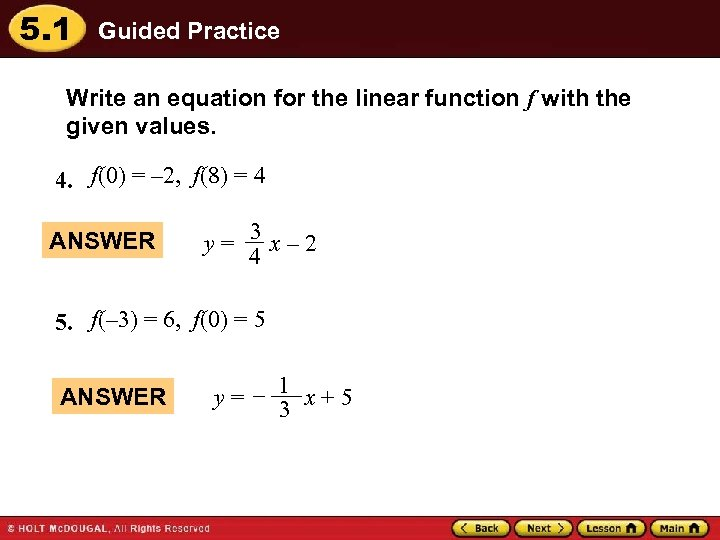5. 1 Guided Practice Write an equation for the linear function f with the given values. 4. f(0) = – 2, f(8) = 4 ANSWER y= 3 x– 2 4 5. f(– 3) = 6, f(0) = 5 ANSWER 1 y= – 3 x+5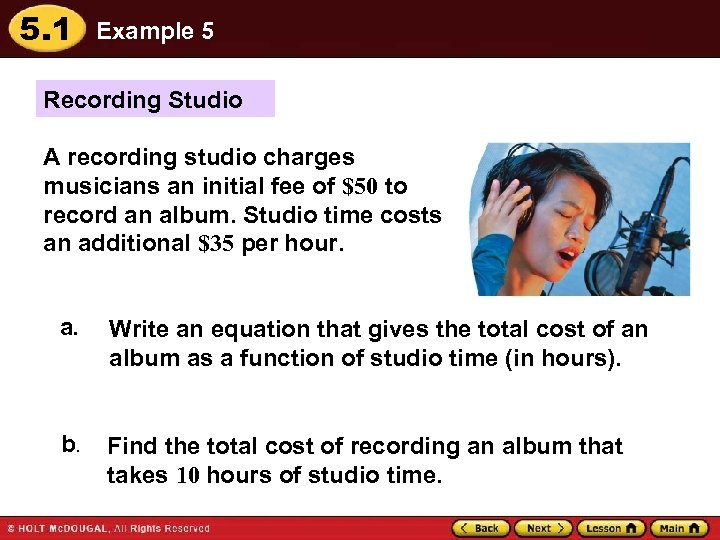5. 1 Example 5 Recording Studio A recording studio charges musicians an initial fee of \$50 to record an album. Studio time costs an additional \$35 per hour. a. Write an equation that gives the total cost of an album as a function of studio time (in hours). b. Find the total cost of recording an album that takes 10 hours of studio time.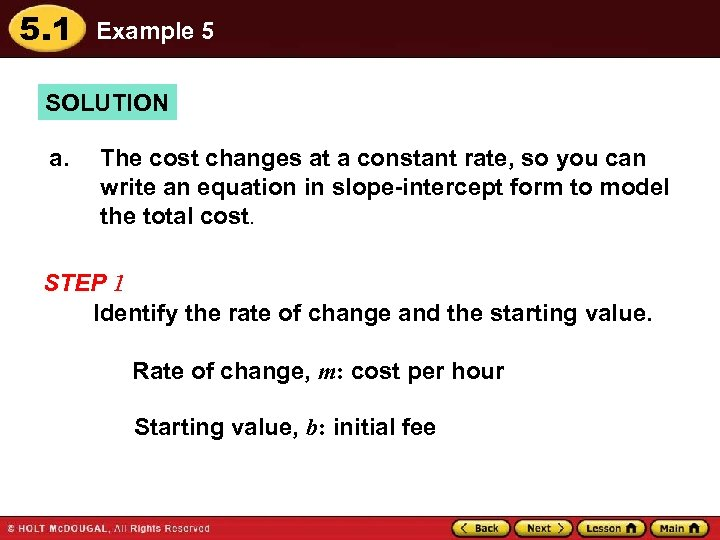5. 1 Example 5 SOLUTION a. The cost changes at a constant rate, so you can write an equation in slope-intercept form to model the total cost. STEP 1 Identify the rate of change and the starting value. Rate of change, m: cost per hour Starting value, b: initial fee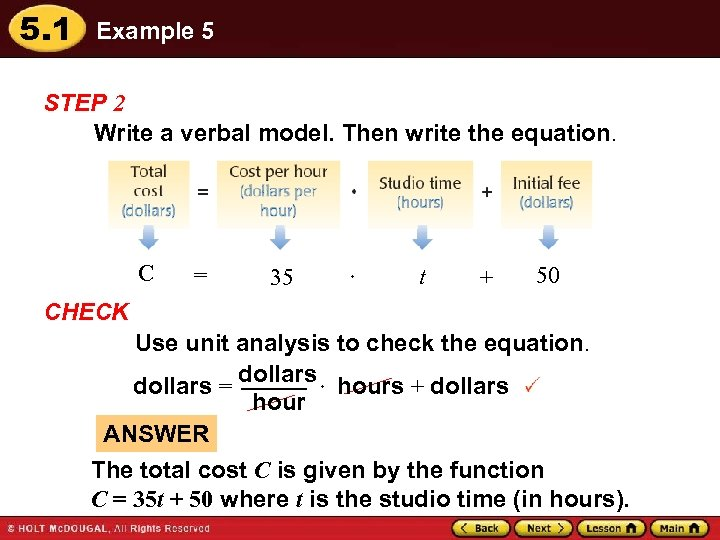5. 1 Example 5 STEP 2 Write a verbal model. Then write the equation. C = 35 t + 50 CHECK Use unit analysis to check the equation. dollars = dollars hours + dollars hour ANSWER The total cost C is given by the function C = 35 t + 50 where t is the studio time (in hours).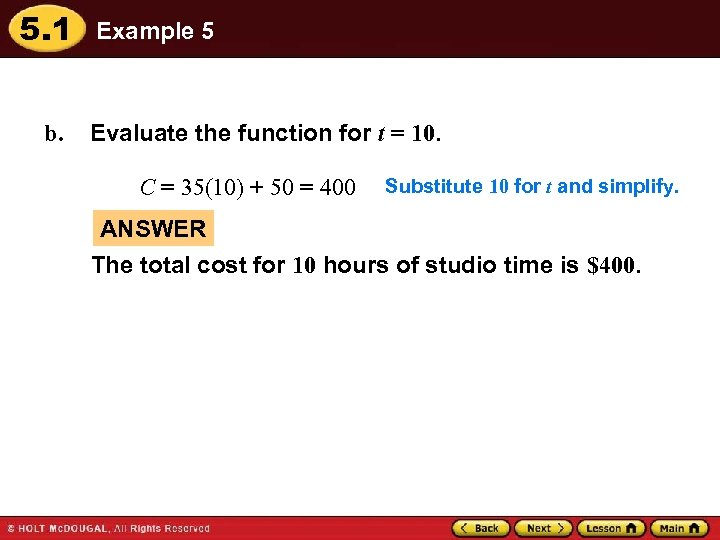5. 1 b. Example 5 Evaluate the function for t = 10. C = 35(10) + 50 = 400 Substitute 10 for t and simplify. ANSWER The total cost for 10 hours of studio time is \$400.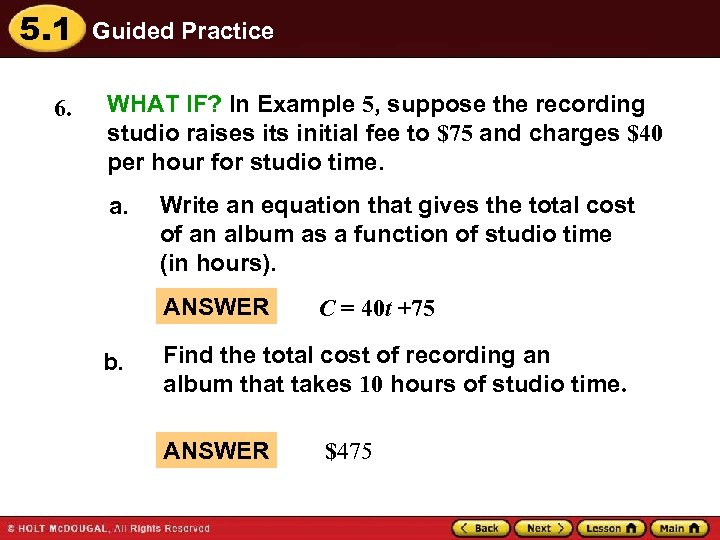5. 1 6. Guided Practice WHAT IF? In Example 5, suppose the recording studio raises its initial fee to \$75 and charges \$40 per hour for studio time. a. Write an equation that gives the total cost of an album as a function of studio time (in hours). ANSWER b. C = 40 t +75 Find the total cost of recording an album that takes 10 hours of studio time. ANSWER \$475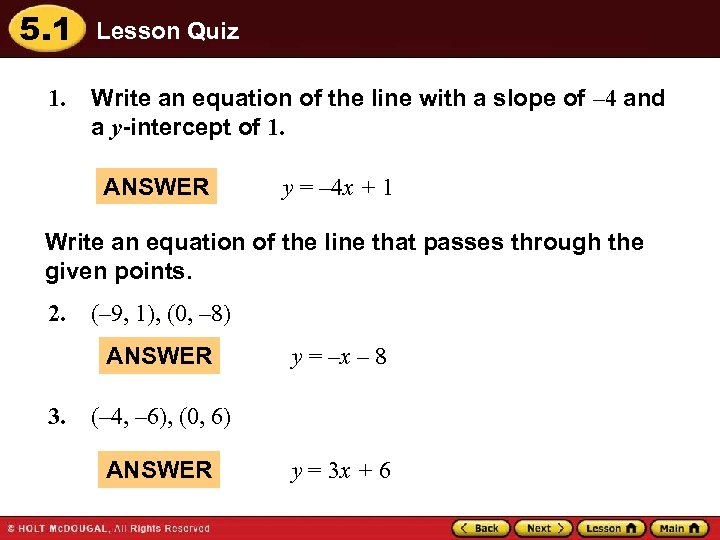5. 1 1. Lesson Quiz Write an equation of the line with a slope of – 4 and a y-intercept of 1. ANSWER y = – 4 x + 1 Write an equation of the line that passes through the given points. 2. (– 9, 1), (0, – 8) ANSWER 3. y = –x – 8 (– 4, – 6), (0, 6) ANSWER y = 3 x + 6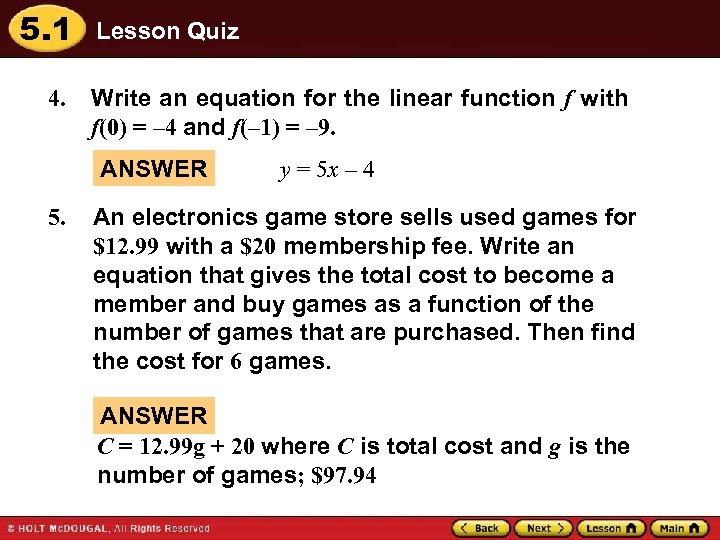5. 1 4. Lesson Quiz Write an equation for the linear function f with f(0) = – 4 and f(– 1) = – 9. ANSWER 5. y = 5 x – 4 An electronics game store sells used games for \$12. 99 with a \$20 membership fee. Write an equation that gives the total cost to become a member and buy games as a function of the number of games that are purchased. Then find the cost for 6 games. ANSWER C = 12. 99 g + 20 where C is total cost and g is the number of games; \$97. 94